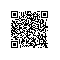# Python 命令行之旅 —— 初探 argparse

Python 命令行之旅 —— 初探 argparse
『讲解开源项目系列』启动——让对开源项目感兴趣的人不再畏惧、让开源项目的发起者不再孤单。跟着我们的文章，你会发现编程的乐趣、使用和发现参与开源项目如此简单。欢迎联系我们给我们投稿，让更多人爱上开源、贡献开源～

argparse 作为 Python 内置的标准库，提供了较为简单的方式来编写命令行接口。当你在程序中定义需要哪些参数，argparse 便会从 sys.argv 中获取命令行输入进行解析，对正确或非法输入做出响应，也可以自动生成帮助信息和使用说明。

import argparse
parser = argparse.ArgumentParser(

description='My Cmd Line Program',

)

# 其类型为 int，且支持输入多个，且至少需要提供一个

                help='a num for the accumulator')

# 若不提供 --sum，默认值为 max 函数，否则为 sum 函数

                const=sum, default=max,
help='sum the nums (default: find the max)')

args = parser.parse_args(['--sum', '-1', '0', '1'])
print(args) # 结果：Namespace(accumulate=, nums=[-1, 0, 1])

result = args.accumulate(args.nums)
print(result) # 基于上文的 ['--sum', '-1', '0', '1'] 参数，accumulate 为 sum 函数，其结果为 0

import argparse

# 1. 设置解析器

parser = argparse.ArgumentParser(

description='My Cmd Line Program',

)

# 2. 定义参数

                help='a num for the accumulator')

                const=sum, default=max,
help='sum the nums (default: find the max)')


# 3. 解析命令行

args = parser.parse_args()

# 4. 业务逻辑

result = args.accumulate(args.nums)
print(result)

$python3 cmd.py --sum -1 0 1 0 若我们需要对一组数字求最大值，只需执行：$ python3 cmd.py -1 0 1
1

\$ python3 cmd.py a b c
usage: cmd.py [-h] [--sum] num [num ...]
cmd.py: error: argument num: invalid int value: 'a'

usage: cmd.py [-h] [--sum] num [num ...]

My Cmd Line Program

positional arguments:
num a num for the accumulator

optional arguments:
-h, --help show this help message and exit
--sum sum the nums (default: find the max)使用钉钉扫一扫加入圈子
+ 订阅